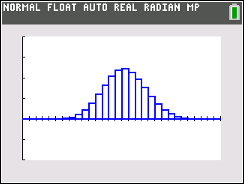# Activities

•• ##### Subject Area

• Math: Statistics: Probability and Random Variables

9-12

50 Minutes

• ##### Device
•TI-84 Plus CE

## Binomial PDF Color#### Activity Overview

This activity involves binomial trials, distributions, and probabilities. Students can create the binomial distribution following the steps in Binomial_Pdf_Eye_Color_Create-84 document.

#### Objectives

Students will:

• Find the probability of specific outcomes from a spreadsheet of values.
• Find the probability of specific outcomes from the graph.
• Find the probability of mutually exclusive events from a graph or spreadsheet.
• Change the sample size and the probability of a success and find the probability of specific outcomes.

#### Vocabulary

• binomial distribution
• expected value
• probability